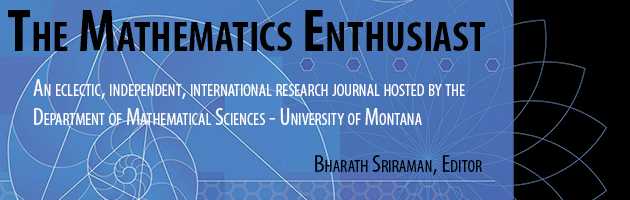•
•#### Article Title

The mathematical continuum: A haunting problematic

15

1-2

#### Abstract

The mathematical continuum has a number of formulations and technical definitions. Two of these reference the geometric line and the real number system. This conceptual coupling of line and number has been an enduring source for mathematical invention and paradox. The continuum captures the monstrous desire of mathematics, a desire to re-assemble the point with the line, the discrete with the continuous, the finite with the infinite. This paper explores how the continuum is a source of fundamental ambiguity fueling our desires and fears about mathematics.

148

158

COinS